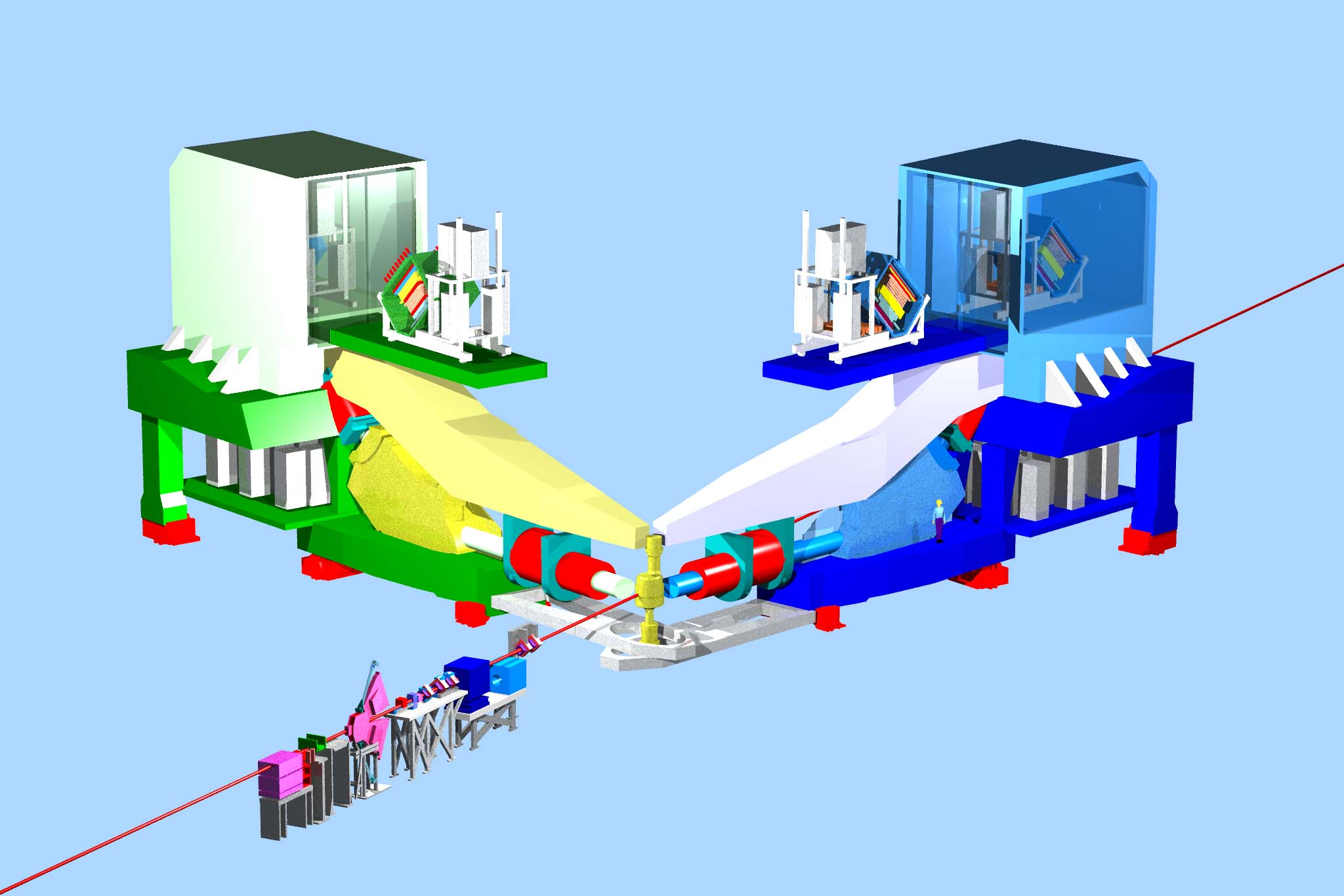Momentum Spectrometer Configuration for PREX/CREX

# PREX/CREX Experiments

The approved and funded PREX experiment at Jefferson Lab will measure the PV asymmetry in the elastic scattering of polarized electrons from $\rm ^{208}Pb$ nucleus to extract a model independent measurement of the neutron skin thickness of the $\rm ^{208}Pb$. A precise measurement of neutron skin thickness helps to constrain the density dependence of the symmetry energy of neutron rich nuclear matter, which has implications on neutron stars, supernova, heavy ion collisions, and atomic parity violation experiments. Recent studies have shown that gravitational wave observations of massive neutron stars during merger provides information on equation of state (EOS). Laboratory measurements like PREX/CREX provides an important feedback on the density dependence of EOS. The CREX experiment measures the neutron skin thickness on $\rm ^{48}Ca$ nuclei. Experimental results from $\rm ^{208}Pb$ and $\rm ^{48}Ca$ will provide a bridge between medium nuclei ab initio calculations and heavy nuclei Density Functional Theory (DFT) calculations over a large range of atomic number (A). An initial measurement for $\rm ^{208}Pb$ skin thickness (PREX-I) was proved statistically limited and motivated to take more data for $\rm ^{208}Pb$ with the next PREX (PREX-II) experiment.